# Antiderivatives, Differential Equations, and Slope FieldsPage 1

#### WATCH ALL SLIDES

Slide 1AP Calculus AB

Antiderivatives,

Differential Equations,

and Slope Fields

Slide 2## Review

Solution

Consider the equation

Slide 3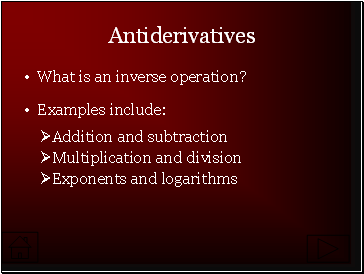## Antiderivatives

What is an inverse operation?

Examples include:

Multiplication and division

Exponents and logariths

Slide 4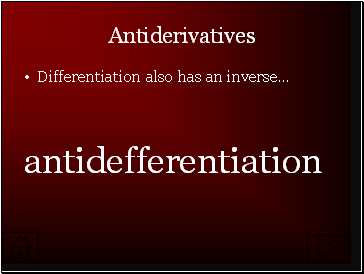Antiderivatives

Differentiation also has an inverse…

antidefferentiation

Slide 5Antiderivatives

Consider the function whose derivative is given by .

What is ?

Solution

We say that is an antiderivative of .

Slide 6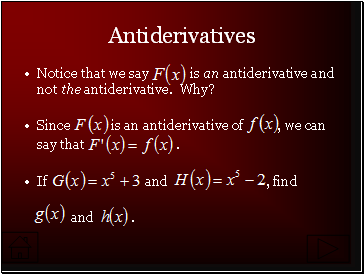Antiderivatives

Notice that we say is an antiderivative and not the antiderivative. Why?

Since is an antiderivative of , we can

say that .

If and , find

and .

Slide 7## Differential Equations

Recall the earlier equation .

This is called a differential equation and could also be written as .

We can think of solving a differential equation as being similar to solving any other equation.

Slide 8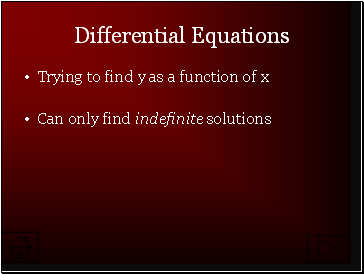Differential Equations

Trying to find y as a function of x

Can only find indefinite solutions

Slide 9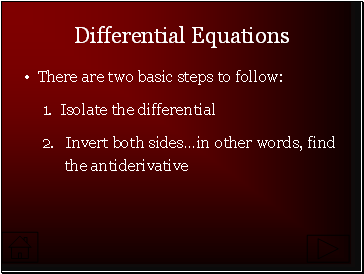Differential Equations

There are two basic steps to follow:

1. Isolate the differential

Invert both sides…in other words, find

the antiderivative

Slide 10Differential Equations

Since we are only finding indefinite solutions, we must indicate the ambiguity of the constant.

Normally, this is done through using a letter to represent any constant. Generally, we use C.

Slide 11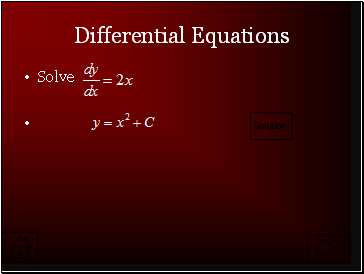Solution

Differential Equations

Solve

Slide 12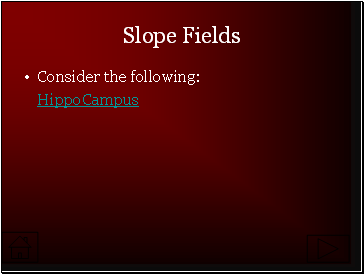## Slope Fields

Consider the following:

HippoCampus

Slide 13Slope Fields

A slope field shows the general “flow” of a differential equation’s solution.

Go to page:
1  2